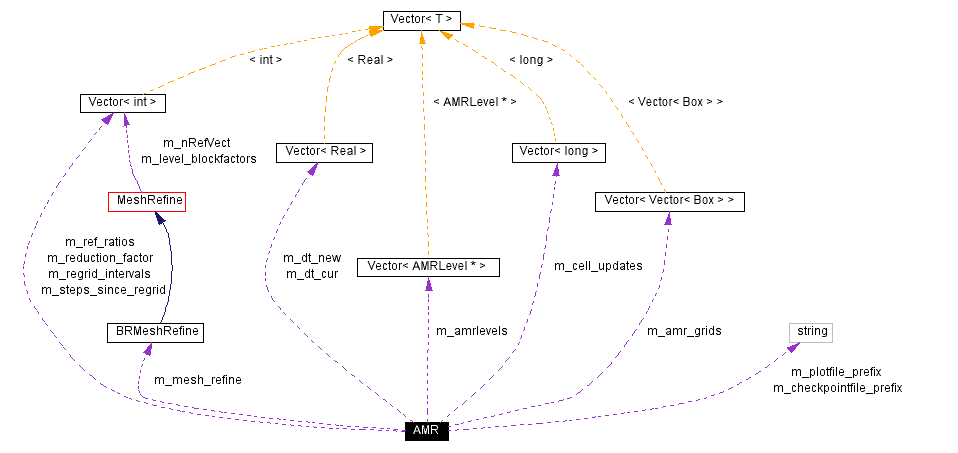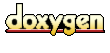Main Page   Namespace List   Class Hierarchy   Alphabetical List   Compound List   File List   Compound Members   File Members

# AMR Class Reference

framework for Berger-Oliger timestepping for AMR More...

#include <AMR.H>

Collaboration diagram for AMR:[legend]
List of all members.

## Public Methods

AMR ()
{\bf constructors, destructors and defines}

~AMR ()
void define (int a_max_level, const Vector< int > &a_ref_ratios, const Box &a_prob_domain, const AMRLevelFactory *const a_amrLevelFact)
void define (int a_max_level, const Vector< int > &a_ref_ratios, const ProblemDomain &a_prob_domain, const AMRLevelFactory *const a_amrLevelFact)
bool isDefined () const
{\bf Setup functions}

bool isSetUp () const
void setupForNewAMRRun ()
void setupForFixedHierarchyRun (const Vector< Vector< Box > > &a_amr_grids, int a_proper_nest=1)
void conclude () const
void run (Real a_max_time, int a_max_step)
void plotPrefix (const std::string &a_plotfile_prefix)

void checkpointPrefix (const std::string &a_checkpointfile_prefix)
void plotInterval (int a_plot_interval)
void checkpointInterval (int a_checkpoint_interval)
void maxGridSize (int a_max_grid_size)
void dtToleranceFactor (Real a_dt_tolerance_factor)
void fillRatio (Real a_fillRat)
void blockFactor (int a_blockFactor)
void gridBufferSize (int a_grid_buffer_size)
int maxGridSize () const
void verbosity (int a_verbosity)
void regridIntervals (const Vector< int > &a_regridIntervals)
int verbosity () const
void maxDtGrow (Real a_dtGrowFactor)
Real maxDtGrow () const
void fixedDt (Real a_dt)
Real fixedDt () const
Vector< AMRLevel * > getAMRLevels ()
Real getCurrentTime () const

## Protected Methods

int timeStep (int a_level, int a_stepsLeft, bool timeBoundary)
void clearMemory ()
void regrid (int a_base_level)
void initialGrid ()
void writePlotFile () const
void writeCheckpointFile () const
void assignDt ()
bool needToRegrid (int a_level, int a_numStepsLeft) const
void makeBaseLevelMesh (Vector< Box > &a_grids) const
void setDefaultValues ()

## Protected Attributes

int m_blockFactor
Real m_fillRatio
int m_max_level
int m_finest_level_old
int m_finest_level
int m_checkpoint_interval
int m_plot_interval
int m_max_grid_size
Real m_dt_tolerance_factor
Real m_fixedDt
bool m_isDefined
bool m_isSetUp
Vector< AMRLevel * > m_amrlevels
Vector< int > m_ref_ratios
Vector< int > m_reduction_factor
Vector< int > m_regrid_intervals
Real m_dt_base
Vector< Realm_dt_new
Vector< Realm_dt_cur
Real m_maxDtGrow
BRMeshRefine m_mesh_refine
bool m_use_meshrefine
Vector< Vector< Box > > m_amr_grids
int m_cur_step
int m_restart_step
int m_lastcheck_step
Real m_cur_time
Vector< int > m_steps_since_regrid
std::string m_plotfile_prefix
std::string m_checkpointfile_prefix
int m_verbosity

## Detailed Description

framework for Berger-Oliger timestepping for AMR

This class is a framework for Berger-Oliger timestepping for adaptive mesh refinement of time-dependent problems. It is applicable to both hyperbolic and parabolic problems. It represents a hierarchy of levels of refinement as a collection of {\tt AMRLevel}s. The usage pattern is this: \ 1. Call define to define the stuff that does not change throughout the run (maxlevel, refinement ratios, domain, and operator) \ 1.5. Modify any parameters you like (blocking factor and so forth using access functions.\ 2. Call any one of the three setup functions so AMR can set up all its internal data structures.\ 3. Call run to run the calculation.\ 4. Call conclude to output (to stdout) how many cells were updated and so forth.

## Constructor & Destructor Documentation

 AMR::AMR ( )
 {\bf constructors, destructors and defines} Default constructor. Use must subsequently call a define() function and a setUp function.

 AMR::~AMR ( )
 Destructor.

## Member Function Documentation

 void AMR::assignDt ( )  [protected]

 void AMR::blockFactor ( int a_blockFactor )
 Set the blocking factor for meshrefinement. Should be called after define and before setup.

 void AMR::checkpointInterval ( int a_checkpoint_interval )
 Sets the interval to write checkpoint files, in terms of the base level time step.

 void AMR::checkpointPrefix ( const std::string & a_checkpointfile_prefix )
 Sets the checkpoint file prefix. Should be called after define and before setup.

 void AMR::clearMemory ( )  [protected]

 void AMR::conclude ( ) const
 You should call this last. It writes the last checkpoint file and tells you how many cells you updated and all that.

 void AMR::define ( int a_max_level, const Vector< int > & a_ref_ratios, const ProblemDomain & a_prob_domain, const AMRLevelFactory *const a_amrLevelFact )
 Defines this object. User must call a setup function before running. {\bf Arguments:}\ a_prob_domain (not modified): problem domain on the base level.\ a_ref_ratios (not modified): refinement ratios. There must be at least a_max_level+1 elements, or an error will result. Element zero is the base level.\ a_max_level (not modified): the maximum level allowed, where the base level is zero. There will be a total of a_max_level+1 levels, since level zero and level a_max_level will both exist.\ a_amrlevel_ptr (not modified): pointer to a physics class factory object. The object it points to is used to construct the collection of AMRLevels in this AMR as objects of the physics class type. It can be destructed after this define() returns.

 void AMR::define ( int a_max_level, const Vector< int > & a_ref_ratios, const Box & a_prob_domain, const AMRLevelFactory *const a_amrLevelFact )
 Defines this object. User must call a setup function before running. {\bf Arguments:}\ a_prob_domain (not modified): problem domain on the base level.\ a_ref_ratios (not modified): refinement ratios. There must be at least a_max_level+1 elements, or an error will result. Element zero is the base level.\ a_max_level (not modified): the maximum level allowed, where the base level is zero. There will be a total of a_max_level+1 levels, since level zero and level a_max_level will both exist.\ a_amrlevel_ptr (not modified): pointer to a physics class factory object. The object it points to is used to construct the collection of AMRLevels in this AMR as objects of the physics class type. It can be destructed after this define() returns.

 void AMR::dtToleranceFactor ( Real a_dt_tolerance_factor )
 Set the factor by which the current dt must exceed the new (max) dt for time subcycling to occur (i.e., reduction of the current dt by powers of 2).

 void AMR::fillRatio ( Real a_fillRat )
 Set the fill ratio for meshrefinement. Should be called after define and before setup.

 Real AMR::fixedDt ( ) const
 Returns the fixed timestep.

 void AMR::fixedDt ( Real a_dt )
 Set a fixed timestep.

 Vector AMR::getAMRLevels ( )

 Real AMR::getCurrentTime ( ) const

 void AMR::gridBufferSize ( int a_grid_buffer_size )
 Set the buffering for the meshrefinement. Should be called after define and before setup.

 void AMR::initialGrid ( )  [protected]

 bool AMR::isDefined ( ) const
 {\bf Setup functions} Has a define function been called? Lots of these functions will assert fail if not.

 bool AMR::isSetUp ( ) const
 Has a setUp function been called? If not, you can't call run

 void AMR::makeBaseLevelMesh ( Vector< Box > & a_grids ) const [protected]

 Real AMR::maxDtGrow ( ) const
 Returns current maximum factor by which a timestep can grow.

 void AMR::maxDtGrow ( Real a_dtGrowFactor )
 Set maximum factor by which a timestep can grow.

 int AMR::maxGridSize ( ) const

 void AMR::maxGridSize ( int a_max_grid_size )
 Set the maximum grid size. Should be called after define and before setup.

 bool AMR::needToRegrid ( int a_level, int a_numStepsLeft ) const [protected]

 void AMR::plotInterval ( int a_plot_interval )
 Sets the interval to write plot files, in terms of the base level time step.

 void AMR::plotPrefix ( const std::string & a_plotfile_prefix )
 {\bf Administrative functions} Sets the plot file prefix. Should be called after define and before setup.

 void AMR::regrid ( int a_base_level )  [protected]

 void AMR::regridIntervals ( const Vector< int > & a_regridIntervals )
 Sets the regridding intervals. This should be OK to call any time after define and before run.

 void AMR::run ( Real a_max_time, int a_max_step )
 Runs the calculation. User must have previously called both the define function and a setup function.

 void AMR::setDefaultValues ( )  [protected]

 void AMR::setupForFixedHierarchyRun ( const Vector< Vector< Box > > & a_amr_grids, int a_proper_nest = 1 )
 This sets the hierarchy and sets regrid_intervals to -1 (turns off regridding). If you want to keep regridding on, reset regridIntervals after this call;

 void AMR::setupForNewAMRRun ( )
 Sets up this object for cold start. User must have previously called define(). Need to call this or setupForRestart or setupforFixedHierarchyRun before you run.

 int AMR::timeStep ( int a_level, int a_stepsLeft, bool timeBoundary )  [protected]

 int AMR::verbosity ( ) const
 Returns current verbosity level. -inf to 0: print nothing. \ 1: prints a message every coarse time step. \ 2: also prints a message when regridding and writing files. \ 3: also prints function trace, and some more inf during file I/O. \ 4 to inf: also prints list of boxes and processor maps during regrid.

 void AMR::verbosity ( int a_verbosity )
 Sets verbosity level to a_verbosity. -inf to 0: print nothing. \ 1: prints a message every coarse time step. \ 2: also prints a message when regridding and writing files. \ 3: also prints function trace, and some more inf during file I/O. \ 4 to inf: also prints list of boxes and processor maps during regrid. This should be OK to call any time after define and before run.

 void AMR::writeCheckpointFile ( ) const [protected]

 void AMR::writePlotFile ( ) const [protected]

## Member Data Documentation

 Vector > AMR::m_amr_grids [protected]

 Vector AMR::m_amrlevels [protected]

 int AMR::m_blockFactor [protected]

 Vector AMR::m_cell_updates [protected]

 int AMR::m_checkpoint_interval [protected]

 std::string AMR::m_checkpointfile_prefix [protected]

 int AMR::m_cur_step [protected]

 Real AMR::m_cur_time [protected]

 Real AMR::m_dt_base [protected]

 Vector AMR::m_dt_cur [protected]

 Vector AMR::m_dt_new [protected]

 Real AMR::m_dt_tolerance_factor [protected]

 Real AMR::m_fillRatio [protected]

 int AMR::m_finest_level [protected]

 int AMR::m_finest_level_old [protected]

 Real AMR::m_fixedDt [protected]

 bool AMR::m_isDefined [protected]

 bool AMR::m_isSetUp [protected]

 int AMR::m_lastcheck_step [protected]

 int AMR::m_max_grid_size [protected]

 int AMR::m_max_level [protected]

 Real AMR::m_maxDtGrow [protected]

 BRMeshRefine AMR::m_mesh_refine [protected]

 int AMR::m_plot_interval [protected]

 std::string AMR::m_plotfile_prefix [protected]

 Vector AMR::m_reduction_factor [protected]

 Vector AMR::m_ref_ratios [protected]

 Vector AMR::m_regrid_intervals [protected]

 int AMR::m_restart_step [protected]

 Vector AMR::m_steps_since_regrid [protected]

 bool AMR::m_use_meshrefine [protected]

 int AMR::m_verbosity [protected]

The documentation for this class was generated from the following file:
Generated on Thu Aug 29 11:07:33 2002 for Chombo&INS by1.2.16